Different Types of Joins in Pandas

• Last Updated : 17 Dec, 2020

The pandas module contains various features to perform various operations on dataframes like join, concatenate, delete, add, etc. In this article, we are going to discuss the various types of join operations that can be performed on pandas dataframe. There are mainly five types of Joins in Pandas:

• Inner Join
• Left Outer Join
• Right Outer Join
• Full Outer Join or simply Outer Join
• Index Join

To understand different types of joins, we will first make two DataFrames, namely a and b.

Dataframe a:

Python3

 # importing pandas  import pandas as pd  # Creating dataframe aa = pd.DataFrame()  # Creating Dictionaryd = {'id': [1, 2, 10, 12],      'val1': ['a', 'b', 'c', 'd']}  a = pd.DataFrame(d)  # printing the dataframea

Output: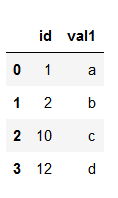DataFrame b:

Python3

 # importing pandasimport pandas as pd  # Creating dataframe bb = pd.DataFrame()  # Creating dictionaryd = {'id': [1, 2, 9, 8],     'val1': ['p', 'q', 'r', 's']}b = pd.DataFrame(d)  # printing the dataframeb

Output: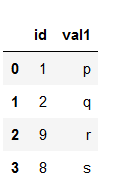We will use these two dataframes to understand the different types of joins.

• Inner Join: Inner join is the most common type of join you’ll be working with. It returns a dataframe with only those rows that have common characteristics. This is similar to the intersection of two sets.Example:

Python3

 # importing pandasimport pandas as pd  # Creating dataframe aa = pd.DataFrame()  # Creating Dictionaryd = {'id': [1, 2, 10, 12],     'val1': ['a', 'b', 'c', 'd']}  a = pd.DataFrame(d)  # Creating dataframe bb = pd.DataFrame()  # Creating dictionaryd = {'id': [1, 2, 9, 8],     'val1': ['p', 'q', 'r', 's']}b = pd.DataFrame(d)  # inner joindf = pd.merge(a, b, on='id', how='inner')  # display dataframedf

Output: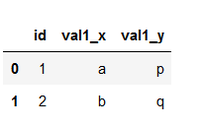• Left Outer Join: With a left outer join, all the records from the first dataframe will be displayed, irrespective of whether the keys in the first dataframe can be found in the second dataframe. Whereas, for the second dataframe, only the records with the keys in the second dataframe that can be found in the first dataframe will be displayed.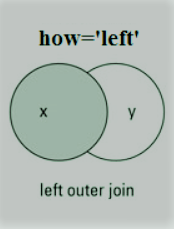Example:

Python3

 # importing pandasimport pandas as pd  # Creating dataframe aa = pd.DataFrame()  # Creating Dictionaryd = {'id': [1, 2, 10, 12],     'val1': ['a', 'b', 'c', 'd']}  a = pd.DataFrame(d)  # Creating dataframe bb = pd.DataFrame()  # Creating dictionaryd = {'id': [1, 2, 9, 8],     'val1': ['p', 'q', 'r', 's']}b = pd.DataFrame(d)  # left outer joindf = pd.merge(a, b, on='id', how='left')  # display dataframedf

Output: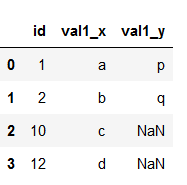• Right Outer Join: For a right join, all the records from the second dataframe will be displayed. However, only the records with the keys in the first dataframe that can be found in the second dataframe will be displayed.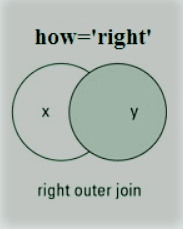Example:

Python3

 # importing pandasimport pandas as pd  # Creating dataframe aa = pd.DataFrame()  # Creating Dictionaryd = {'id': [1, 2, 10, 12],     'val1': ['a', 'b', 'c', 'd']}  a = pd.DataFrame(d)  # Creating dataframe bb = pd.DataFrame()  # Creating dictionaryd = {'id': [1, 2, 9, 8],     'val1': ['p', 'q', 'r', 's']}b = pd.DataFrame(d)  # right outer joindf = pd.merge(a, b, on='id', how='right')  # display dataframedf

Output: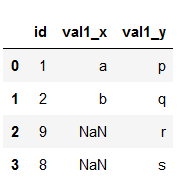• Full Outer Join: A full outer join returns all the rows from the left dataframe, all the rows from the right dataframe, and matches up rows where possible, with NaNs elsewhere. But if the dataframe is complete, then we get the same output.Example:

Python3

 # importing pandasimport pandas as pd  # Creating dataframe aa = pd.DataFrame()  # Creating Dictionaryd = {'id': [1, 2, 10, 12],     'val1': ['a', 'b', 'c', 'd']}  a = pd.DataFrame(d)  # Creating dataframe bb = pd.DataFrame()  # Creating dictionaryd = {'id': [1, 2, 9, 8],     'val1': ['p', 'q', 'r', 's']}b = pd.DataFrame(d)  # full outer joindf = pd.merge(a, b, on='id', how='outer')  # display dataframedf

Output: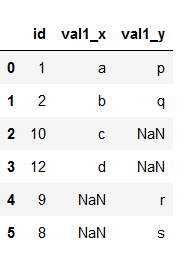• Index Join: To merge the dataframe on indices pass the left_index and right_index arguments as True i.e. both the dataframes are merged on an index using default Inner Join.

Example:

Python3

 # importing pandasimport pandas as pd  # Creating dataframe aa = pd.DataFrame()  # Creating Dictionaryd = {'id': [1, 2, 10, 12],     'val1': ['a', 'b', 'c', 'd']}  a = pd.DataFrame(d)  # Creating dataframe bb = pd.DataFrame()  # Creating dictionaryd = {'id': [1, 2, 9, 8],     'val1': ['p', 'q', 'r', 's']}b = pd.DataFrame(d)  # index joindf = pd.merge(a, b, left_index=True, right_index=True)  # display dataframedf

Output: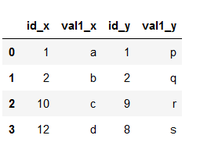My Personal Notes arrow_drop_up